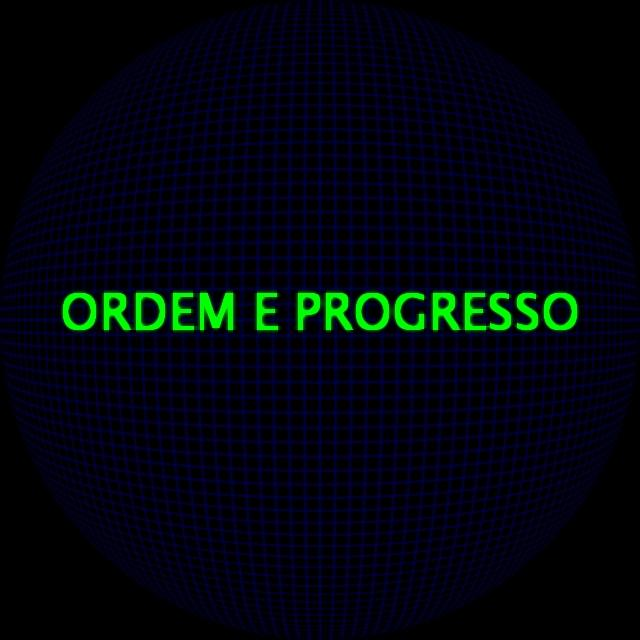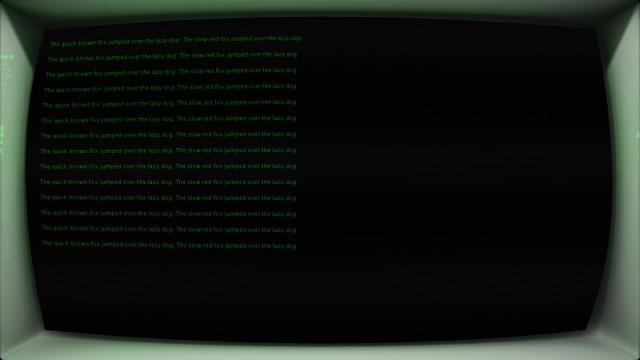#### Howdy, Stranger!

We are about to switch to a new forum software. Until then we have removed the registration on this forum.

# 2 PGraphics and 2 Shaders

edited February 2014

There are 2 PGraphics: canvas and scene

There are 2 Shaders: fisheye and glow

How to apply "fisheye" on canvas and scene and "glow" only on scene (glowing deformed text) ?

processing-2.0b8

``````PShader fisheye, glow;
PGraphics canvas, scene;

void setup() {
size(640, 640, P2D);
frameRate(100);
canvas = createGraphics(width, height, P2D);
scene = createGraphics(width, height, P2D);

fisheye.set("aperture", 180.0);
glow.set("iResolution", float(width), float(height));

}

void draw() {

canvas.beginDraw();
canvas.background(0);
canvas.stroke(0, 0, 100);
for (int i = 0; i < width; i += 10) {
canvas.line(i, 0, i, height);
}
for (int i = 0; i < height; i += 10) {
canvas.line(0, i, width, i);
}
canvas.endDraw();

scene.beginDraw();
scene.background(0, 0);
scene.textSize(50);
scene.fill(0, 255, 0);
scene.text("ORDEM E PROGRESSO", 60, 330);
scene.endDraw();

image(canvas, 0, 0, width, height);
image(scene, 0, 0, width, height);

}
``````

FishEye.glsl

``````#ifdef GL_ES
precision mediump float;
precision mediump int;
#endif

uniform sampler2D texture;
uniform mat4 texMatrix;

varying vec4 vertColor;
varying vec4 vertTexCoord;

uniform float aperture;

const float PI = 3.1415926535;

void main(void) {
float apertureHalf = 0.5 * aperture * (PI / 180.0);

float maxFactor = sin(apertureHalf);

vec2 stFactor = vec2(1.0 / abs(texMatrix), 1.0 / abs(texMatrix));
vec2 pos = (2.0 * vertTexCoord.st * stFactor - 1.0);

float l = length(pos);
if (l > 1.0) {
gl_FragColor = vec4(0, 0, 0, 1);
} else {
float x = maxFactor * pos.x;
float y = maxFactor * pos.y;

float n = length(vec2(x, y));

float z = sqrt(1.0 - n * n);

float r = atan(n, z) / PI;

float phi = atan(y, x);

float u = r * cos(phi) + 0.5;
float v = r * sin(phi) + 0.5;

gl_FragColor = texture2D(texture, vec2(u, v) / stFactor) * vertColor;
}
}
``````

Glow3.glsl

``````#ifdef GL_ES
precision highp float;
#endif

uniform float iGlobalTime;
uniform vec2 iResolution;
uniform sampler2D iChannel0;

const float blurSize = 1.0/512.0;
const float intensity = 1.3;
void main()
{
vec4 sum = vec4(0);
vec2 texcoord = gl_FragCoord.xy/iResolution.xy;
int j;
int i;

sum += texture2D(iChannel0, vec2(texcoord.x - 4.0*blurSize, texcoord.y)) * 0.05;
sum += texture2D(iChannel0, vec2(texcoord.x - 3.0*blurSize, texcoord.y)) * 0.09;
sum += texture2D(iChannel0, vec2(texcoord.x - 2.0*blurSize, texcoord.y)) * 0.12;
sum += texture2D(iChannel0, vec2(texcoord.x - blurSize, texcoord.y)) * 0.15;
sum += texture2D(iChannel0, vec2(texcoord.x, texcoord.y)) * 0.16;
sum += texture2D(iChannel0, vec2(texcoord.x + blurSize, texcoord.y)) * 0.15;
sum += texture2D(iChannel0, vec2(texcoord.x + 2.0*blurSize, texcoord.y)) * 0.12;
sum += texture2D(iChannel0, vec2(texcoord.x + 3.0*blurSize, texcoord.y)) * 0.09;
sum += texture2D(iChannel0, vec2(texcoord.x + 4.0*blurSize, texcoord.y)) * 0.05;

sum += texture2D(iChannel0, vec2(texcoord.x, texcoord.y - 4.0*blurSize)) * 0.05;
sum += texture2D(iChannel0, vec2(texcoord.x, texcoord.y - 3.0*blurSize)) * 0.09;
sum += texture2D(iChannel0, vec2(texcoord.x, texcoord.y - 2.0*blurSize)) * 0.12;
sum += texture2D(iChannel0, vec2(texcoord.x, texcoord.y - blurSize)) * 0.15;
sum += texture2D(iChannel0, vec2(texcoord.x, texcoord.y)) * 0.16;
sum += texture2D(iChannel0, vec2(texcoord.x, texcoord.y + blurSize)) * 0.15;
sum += texture2D(iChannel0, vec2(texcoord.x, texcoord.y + 2.0*blurSize)) * 0.12;
sum += texture2D(iChannel0, vec2(texcoord.x, texcoord.y + 3.0*blurSize)) * 0.09;
sum += texture2D(iChannel0, vec2(texcoord.x, texcoord.y + 4.0*blurSize)) * 0.05;

//increase blur with intensity!
gl_FragColor = sum*intensity + texture2D(iChannel0, texcoord);

}
``````
Tagged:

• edited February 2014
1. In Glow3.glsl change iChannel0 to texture.
2. In your main sketch use:

``````  shader(fisheye);
image(canvas, 0, 0, width, height);
image(scene, 0, 0, width, height);
``````

EDIT Wait, just reread your question. So you want the scene to be both fisheye'd and glow-y. Hmm, indeed tricky. Ideally, you would be able to apply the shader to a PGraphics directly (believe I read somewhere on GitHub that this in development or someone asked a question about it?). Anyway, perhaps you could use a second PGraphics and apply the first shader inside the PGraphics and the second shader on the PGraphics as a whole (as you are doing in your current sketch)?

• edited February 2014

I have tried, but now the text is not deformed.

``````//Glow3.glsl

uniform float iGlobalTime;
uniform vec2 iResolution;
uniform sampler2D texture;

const float blurSize = 1.0/512.0;
const float intensity = 1.3;
void main()
{
vec4 sum = vec4(0);
vec2 texcoord = gl_FragCoord.xy/iResolution.xy;

sum += texture2D(texture, vec2(texcoord.x - 4.0*blurSize, texcoord.y)) * 0.05;
sum += texture2D(texture, vec2(texcoord.x - 3.0*blurSize, texcoord.y)) * 0.09;
sum += texture2D(texture, vec2(texcoord.x - 2.0*blurSize, texcoord.y)) * 0.12;
sum += texture2D(texture, vec2(texcoord.x - blurSize, texcoord.y)) * 0.15;
sum += texture2D(texture, vec2(texcoord.x, texcoord.y)) * 0.16;
sum += texture2D(texture, vec2(texcoord.x + blurSize, texcoord.y)) * 0.15;
sum += texture2D(texture, vec2(texcoord.x + 2.0*blurSize, texcoord.y)) * 0.12;
sum += texture2D(texture, vec2(texcoord.x + 3.0*blurSize, texcoord.y)) * 0.09;
sum += texture2D(texture, vec2(texcoord.x + 4.0*blurSize, texcoord.y)) * 0.05;

sum += texture2D(texture, vec2(texcoord.x, texcoord.y - 4.0*blurSize)) * 0.05;
sum += texture2D(texture, vec2(texcoord.x, texcoord.y - 3.0*blurSize)) * 0.09;
sum += texture2D(texture, vec2(texcoord.x, texcoord.y - 2.0*blurSize)) * 0.12;
sum += texture2D(texture, vec2(texcoord.x, texcoord.y - blurSize)) * 0.15;
sum += texture2D(texture, vec2(texcoord.x, texcoord.y)) * 0.16;
sum += texture2D(texture, vec2(texcoord.x, texcoord.y + blurSize)) * 0.15;
sum += texture2D(texture, vec2(texcoord.x, texcoord.y + 2.0*blurSize)) * 0.12;
sum += texture2D(texture, vec2(texcoord.x, texcoord.y + 3.0*blurSize)) * 0.09;
sum += texture2D(texture, vec2(texcoord.x, texcoord.y + 4.0*blurSize)) * 0.05;

gl_FragColor = sum*intensity + texture2D(texture, texcoord);

}
``````
• deform without glowglow without deform• edited February 2014

btw, I need vintage terminal deformed text. If someone has better idea for glowy shader, let me know!

• edited February 2014

I have tried also apply "glow" shader inside the scene PGraphics, but the result is same as on the image1.

``````  scene.beginDraw();
scene.background(0, 0);
scene.textSize(50);
scene.fill(0, 255, 0);
scene.text("ORDEM E PROGRESSO", 60, 330);
scene.endDraw();

image(canvas, 0, 0, width, height);
image(scene, 0, 0, width, height);
``````
• edited February 2014

vintage terminal (in progress...)• If you want to apply a shader on an offscreen PGraphics, you should call shader/resetShader/etc on the PGraphics, otherwise you are changing the shaders on the main surface and not affecting the offscreen rendering at all:

``````scene.beginDraw();
scene.background(0, 0);
scene.textSize(50);
scene.fill(0, 255, 0);
scene.text("ORDEM E PROGRESSO", 60, 330);
scene.endDraw();
``````

But I think this won't as expected either, because the glow shader is meant to operate the entire scene texture. By using shader in the way above, it will be applied only on the text (text is rendered as images), and not on the grid. A possible solution would be to use the filter() function on the PGraphics after you have drawn the entire offscreen scene:

``````void draw() {
canvas.beginDraw();
canvas.background(0);
canvas.stroke(0, 0, 100);
for (int i = 0; i < width; i += 10) {
canvas.line(i, 0, i, height);
}
for (int i = 0; i < height; i += 10) {
canvas.line(0, i, width, i);
}
canvas.endDraw();

scene.beginDraw();
scene.background(0, 0);
scene.textSize(50);
scene.fill(0, 255, 0);
scene.text("ORDEM E PROGRESSO", 60, 330);
scene.filter(glow);
scene.endDraw();

•